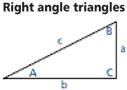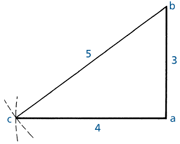# COP v3.0:useful-information; geometry-and-mensuration

## Code of Practice v3.0 Online

The NZ Metal Roof and Wall Cladding Code of Practice is a comprehensive design & installation guide, and a recognised related document for Acceptable Solution E2/AS1 of the NZ Building Code.

## 18.5 Geometry And Mensuration

Enter values below for automatic calculations

= area
= base
= diameter
= height
= length
= 3.1416
= circumference = 2πr or 22/7d

### Areas

= πr² or 0.7854 d²
= bh
= .5 bh
= .5 two parallel sides x h
= 0.8862 d
= 1.1284 side of square
= .66 bh
= 0.7854 d1 d2
Area of any figure of four or more unequal sides is found by dividing it into triangles, finding areas of each and adding together.

### Surface Area

= 6b²
= πd²
= .5 cbh (slant height)
= πdh
= πdh + 2πr2
= ab + c of base x .5h (slant height)

= b³
= 0.5236 d³
= .33 abh
= .33 abh
= πr²h

### Table of polygons

= side of polygon.
= Angle formed by the intersection of the sides.
NameNo of sidesAngle
Trigon360°
Pentagon5108°
Hexagon6120°
Octagon8135°
Decagon10144°
Area of any regular polygon = Radius of inscribed circle x 1/2 number of sides x length of one side.

### Right Angle Triangles

Enter any two values on the illustration for live calculations.

°
1 in

### 18.5A Triangle ValuesFindGiven Solution
A a, b tan A = a / b
a, c sin A = a /c
b, c cos A = b / c
B a, b tan B = b / a
a, c cos B = a / c
b, c sin B = b / c
a A, b b tan A
A, c c sin A
b, c √ c² - b²
b A, a a / tan A
A, c c / cos A
a, c √ c² - a²
c A, a a / sin A
A, b b / cos A
a, b √ a² + b²
Area a, b ab / ²

### 18.5B To Find a Right Angle

Draw a line ab 3x long. At point a scribe an arc 4x long.
At point b scribe an arc 5x long to intersect a c.
Join ac and b, ac and ab are at 900.